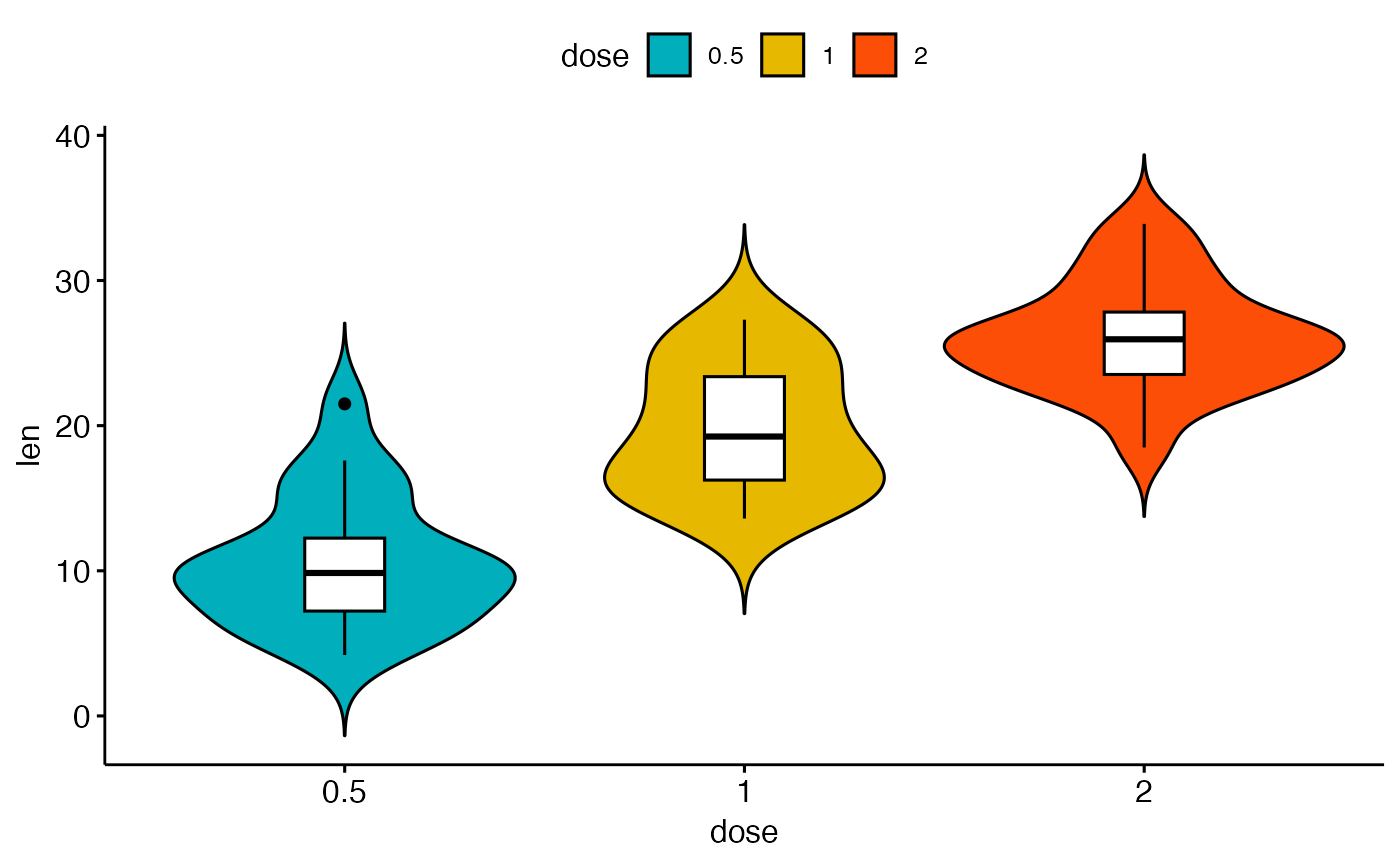Create a violin plot with error bars. Violin plots are similar to box plots, except that they also show the kernel probability density of the data at different values.

ggviolin(
data,
x,
y,
combine = FALSE,
merge = FALSE,
color = "black",
fill = "white",
palette = NULL,
alpha = 1,
title = NULL,
xlab = NULL,
ylab = NULL,
facet.by = NULL,
panel.labs = NULL,
short.panel.labs = TRUE,
linetype = "solid",
trim = FALSE,
size = NULL,
width = 1,
draw_quantiles = NULL,
select = NULL,
remove = NULL,
order = NULL,
error.plot = "pointrange",
label = NULL,
font.label = list(size = 11, color = "black"),
label.select = NULL,
repel = FALSE,
label.rectangle = FALSE,
position = position_dodge(0.8),
ggtheme = theme_pubr(),
...
)

## Arguments

data

a data frame

x

character string containing the name of x variable.

y

character vector containing one or more variables to plot

combine

logical value. Default is FALSE. Used only when y is a vector containing multiple variables to plot. If TRUE, create a multi-panel plot by combining the plot of y variables.

merge

logical or character value. Default is FALSE. Used only when y is a vector containing multiple variables to plot. If TRUE, merge multiple y variables in the same plotting area. Allowed values include also "asis" (TRUE) and "flip". If merge = "flip", then y variables are used as x tick labels and the x variable is used as grouping variable.

color

outline color.

fill

fill color.

palette

the color palette to be used for coloring or filling by groups. Allowed values include "grey" for grey color palettes; brewer palettes e.g. "RdBu", "Blues", ...; or custom color palette e.g. c("blue", "red"); and scientific journal palettes from ggsci R package, e.g.: "npg", "aaas", "lancet", "jco", "ucscgb", "uchicago", "simpsons" and "rickandmorty".

alpha

color transparency. Values should be between 0 and 1.

title

plot main title.

xlab

character vector specifying x axis labels. Use xlab = FALSE to hide xlab.

ylab

character vector specifying y axis labels. Use ylab = FALSE to hide ylab.

facet.by

character vector, of length 1 or 2, specifying grouping variables for faceting the plot into multiple panels. Should be in the data.

panel.labs

a list of one or two character vectors to modify facet panel labels. For example, panel.labs = list(sex = c("Male", "Female")) specifies the labels for the "sex" variable. For two grouping variables, you can use for example panel.labs = list(sex = c("Male", "Female"), rx = c("Obs", "Lev", "Lev2") ).

short.panel.labs

logical value. Default is TRUE. If TRUE, create short labels for panels by omitting variable names; in other words panels will be labelled only by variable grouping levels.

linetype

line types.

trim

If TRUE (default), trim the tails of the violins to the range of the data. If FALSE, don't trim the tails.

size

Numeric value (e.g.: size = 1). change the size of points and outlines.

width

violin width.

draw_quantiles

If not(NULL) (default), draw horizontal lines at the given quantiles of the density estimate.

select

character vector specifying which items to display.

remove

character vector specifying which items to remove from the plot.

order

character vector specifying the order of items.

character vector for adding another plot element (e.g.: dot plot or error bars). Allowed values are one or the combination of: "none", "dotplot", "jitter", "boxplot", "point", "mean", "mean_se", "mean_sd", "mean_ci", "mean_range", "median", "median_iqr", "median_hilow", "median_q1q3", "median_mad", "median_range"; see ?desc_statby for more details.

parameters (color, shape, size, fill, linetype) for the argument 'add'; e.g.: add.params = list(color = "red").

error.plot

plot type used to visualize error. Allowed values are one of c("pointrange", "linerange", "crossbar", "errorbar", "upper_errorbar", "lower_errorbar", "upper_pointrange", "lower_pointrange", "upper_linerange", "lower_linerange"). Default value is "pointrange" or "errorbar". Used only when add != "none" and add contains one "mean_*" or "med_*" where "*" = sd, se, ....

label

the name of the column containing point labels. Can be also a character vector with length = nrow(data).

font.label

a list which can contain the combination of the following elements: the size (e.g.: 14), the style (e.g.: "plain", "bold", "italic", "bold.italic") and the color (e.g.: "red") of labels. For example font.label = list(size = 14, face = "bold", color ="red"). To specify only the size and the style, use font.label = list(size = 14, face = "plain").

label.select

can be of two formats:

• a character vector specifying some labels to show.

• a list containing one or the combination of the following components:

• top.up and top.down: to display the labels of the top up/down points. For example, label.select = list(top.up = 10, top.down = 4).

• criteria: to filter, for example, by x and y variabes values, use this: label.select = list(criteria = "y > 2 & y < 5 & x %in% c('A', 'B')").

repel

a logical value, whether to use ggrepel to avoid overplotting text labels or not.

label.rectangle

logical value. If TRUE, add rectangle underneath the text, making it easier to read.

position

Position adjustment, either as a string naming the adjustment (e.g. "jitter" to use position_jitter), or the result of a call to a position adjustment function. Use the latter if you need to change the settings of the adjustment.

ggtheme

function, ggplot2 theme name. Default value is theme_pubr(). Allowed values include ggplot2 official themes: theme_gray(), theme_bw(), theme_minimal(), theme_classic(), theme_void(), ....

...

other arguments to be passed to geom_violin, ggpar and facet.

## Details

The plot can be easily customized using the function ggpar(). Read ?ggpar for changing:

• main title and axis labels: main, xlab, ylab

• axis limits: xlim, ylim (e.g.: ylim = c(0, 30))

• axis scales: xscale, yscale (e.g.: yscale = "log2")

• color palettes: palette = "Dark2" or palette = c("gray", "blue", "red")

• legend title, labels and position: legend = "right"

• plot orientation : orientation = c("vertical", "horizontal", "reverse")

ggpar

## Examples

# Load data
data("ToothGrowth")
df <- ToothGrowth

# Basic plot
# +++++++++++++++++++++++++++
ggviolin(df, x = "dose", y = "len")# Change the plot orientation: horizontal
ggviolin(df, "dose", "len", orientation = "horiz")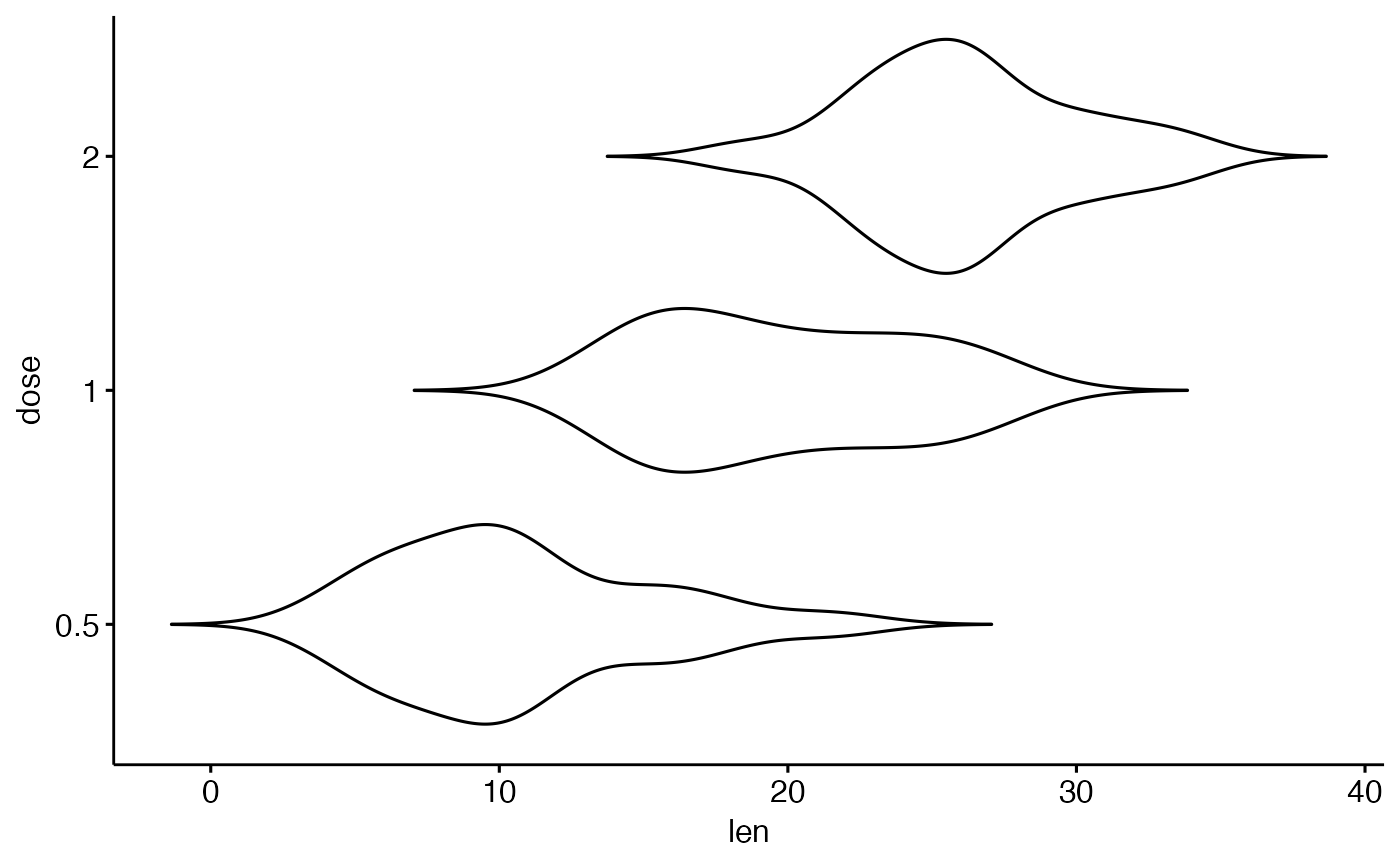# ++++++++++++++++++++++++++
# Draw quantiles
ggviolin(df, "dose", "len", add = "none",
draw_quantiles = 0.5)ggviolin(df, x = "dose", y = "len",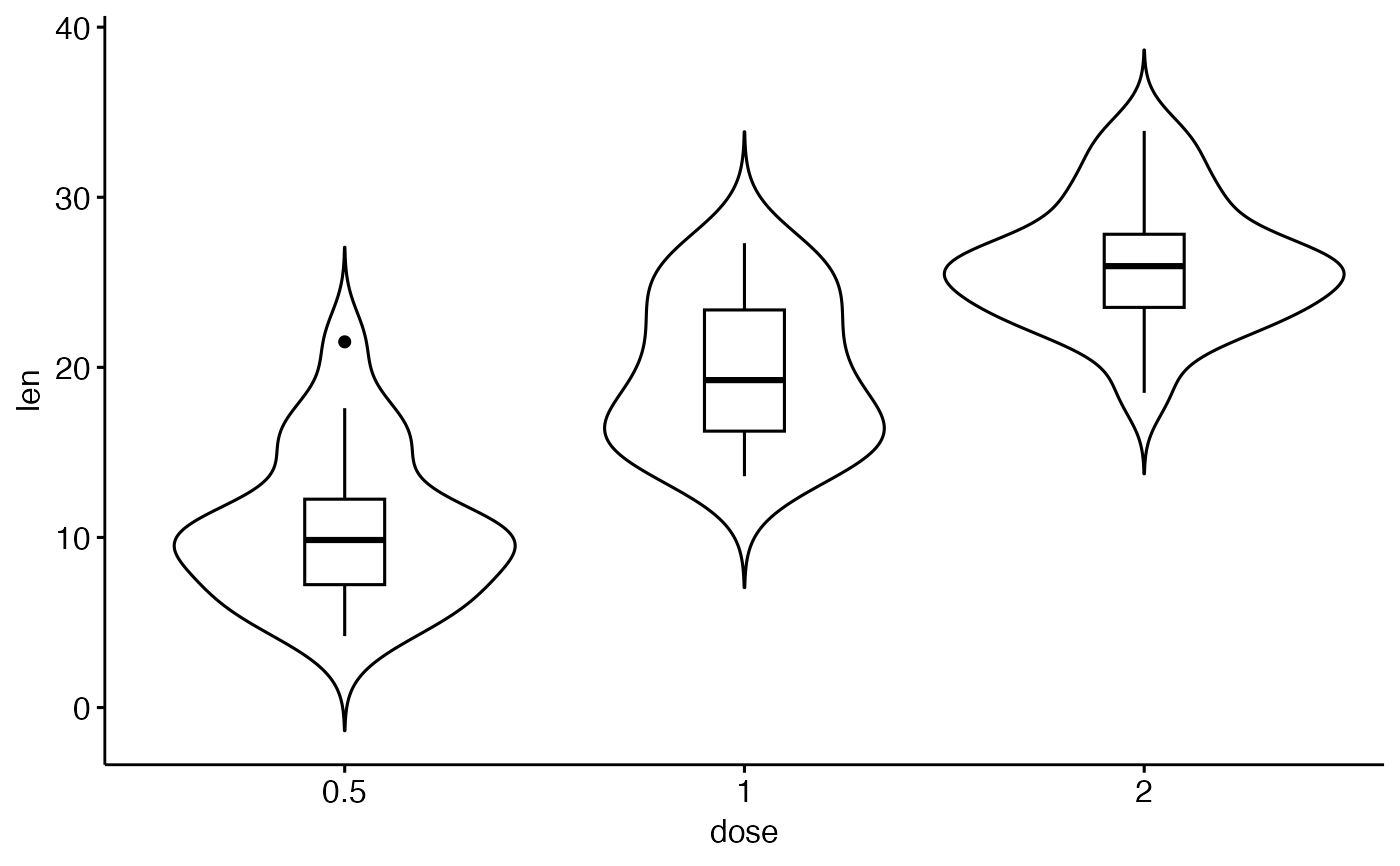ggviolin(df, x = "dose", y = "len",
#> Bin width defaults to 1/30 of the range of the data. Pick better value with
#> binwidth.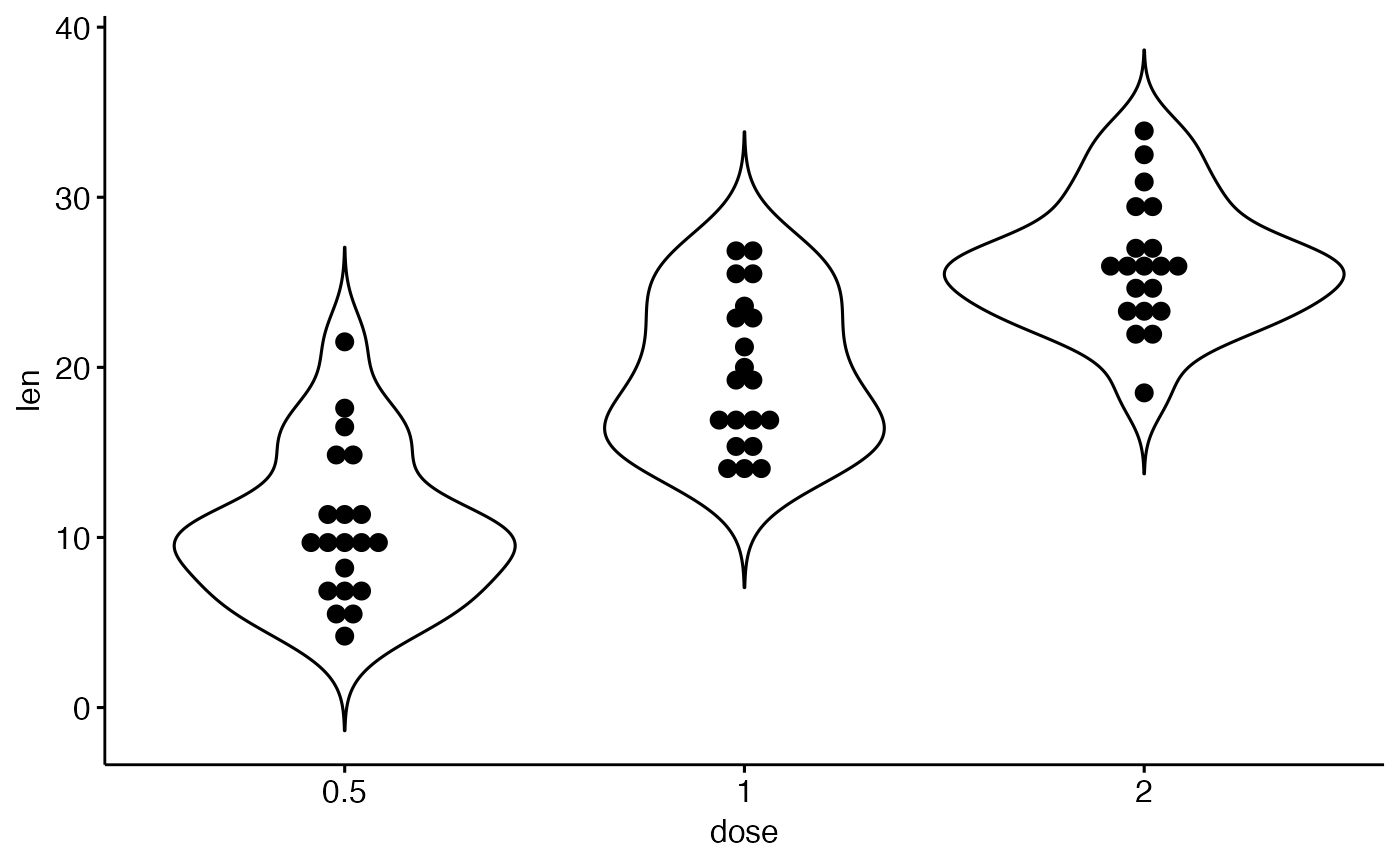# change point shape by groups ("dose")
ggviolin(df, x = "dose", y = "len",
add = "jitter", shape = "dose")# Add mean_sd + jittered points
ggviolin(df, x = "dose", y = "len",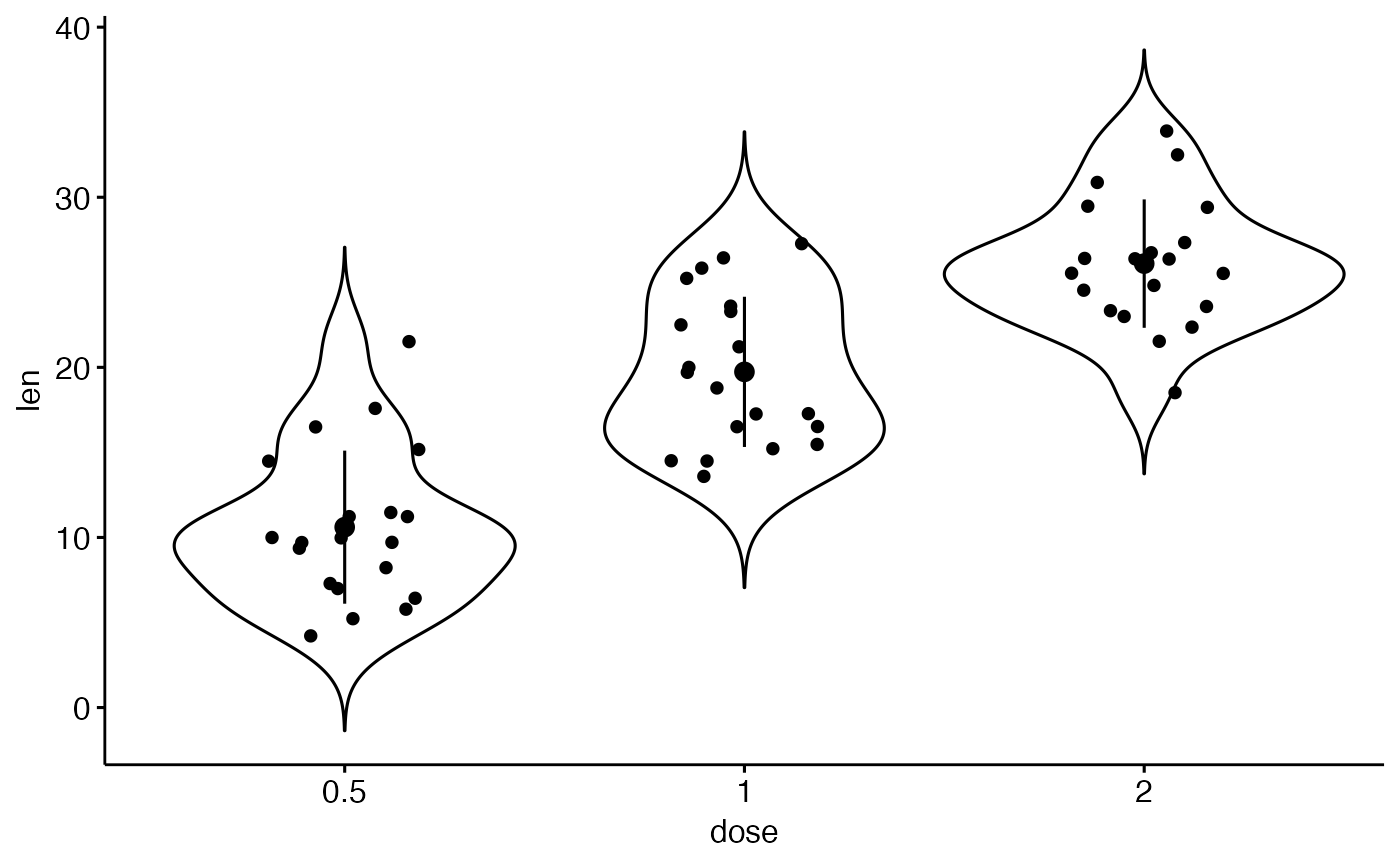# Change error.plot to "crossbar"
ggviolin(df, x = "dose", y = "len",
add = "mean_sd", error.plot = "crossbar")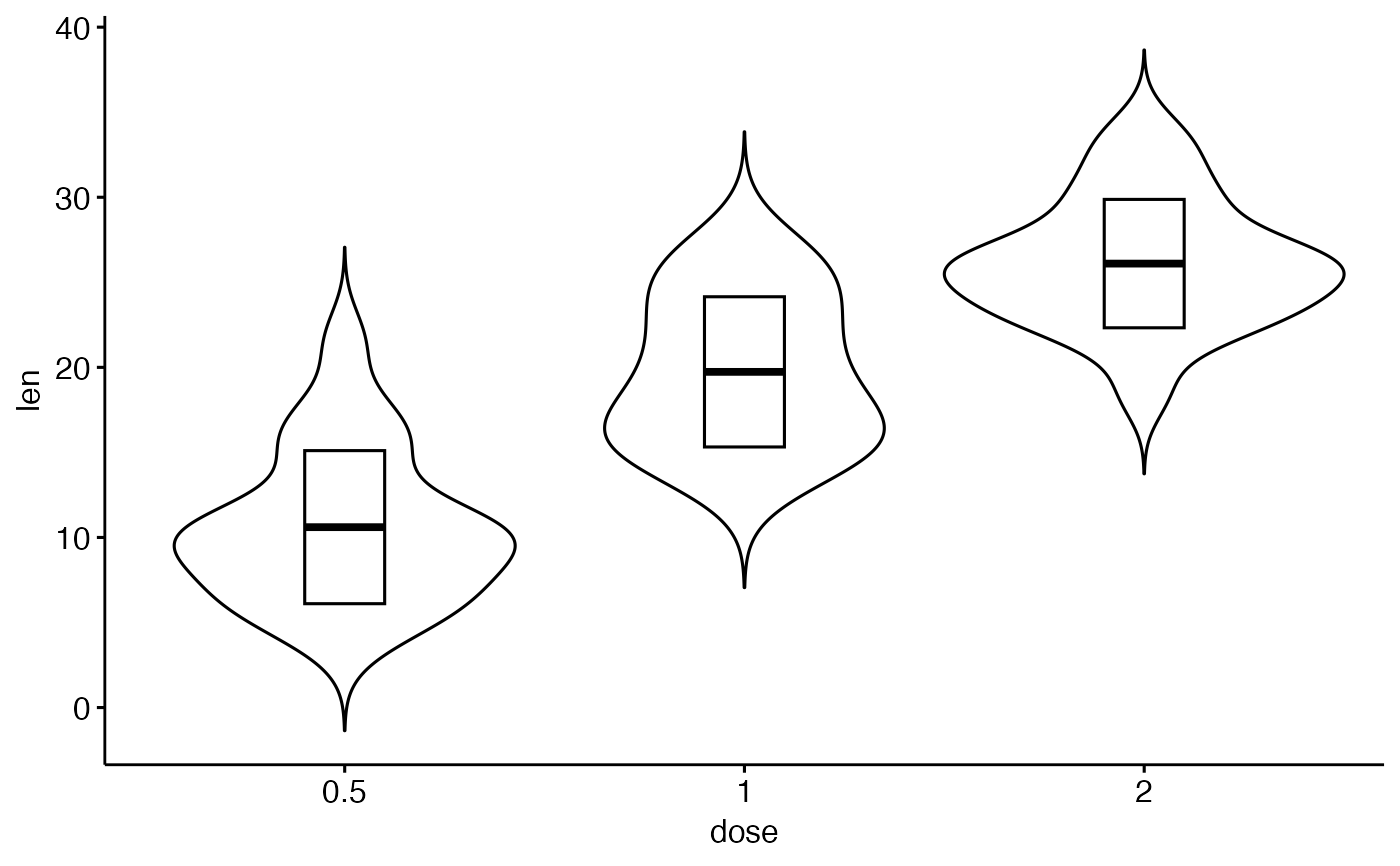# Change colors
# +++++++++++++++++++++++++++
# Change outline and fill colors
ggviolin(df, "dose", "len",
color = "black", fill = "gray")# Change outline colors by groups: dose
# Use custom color palette and add boxplot
ggviolin(df, "dose", "len",  color = "dose",
palette = c("#00AFBB", "#E7B800", "#FC4E07"),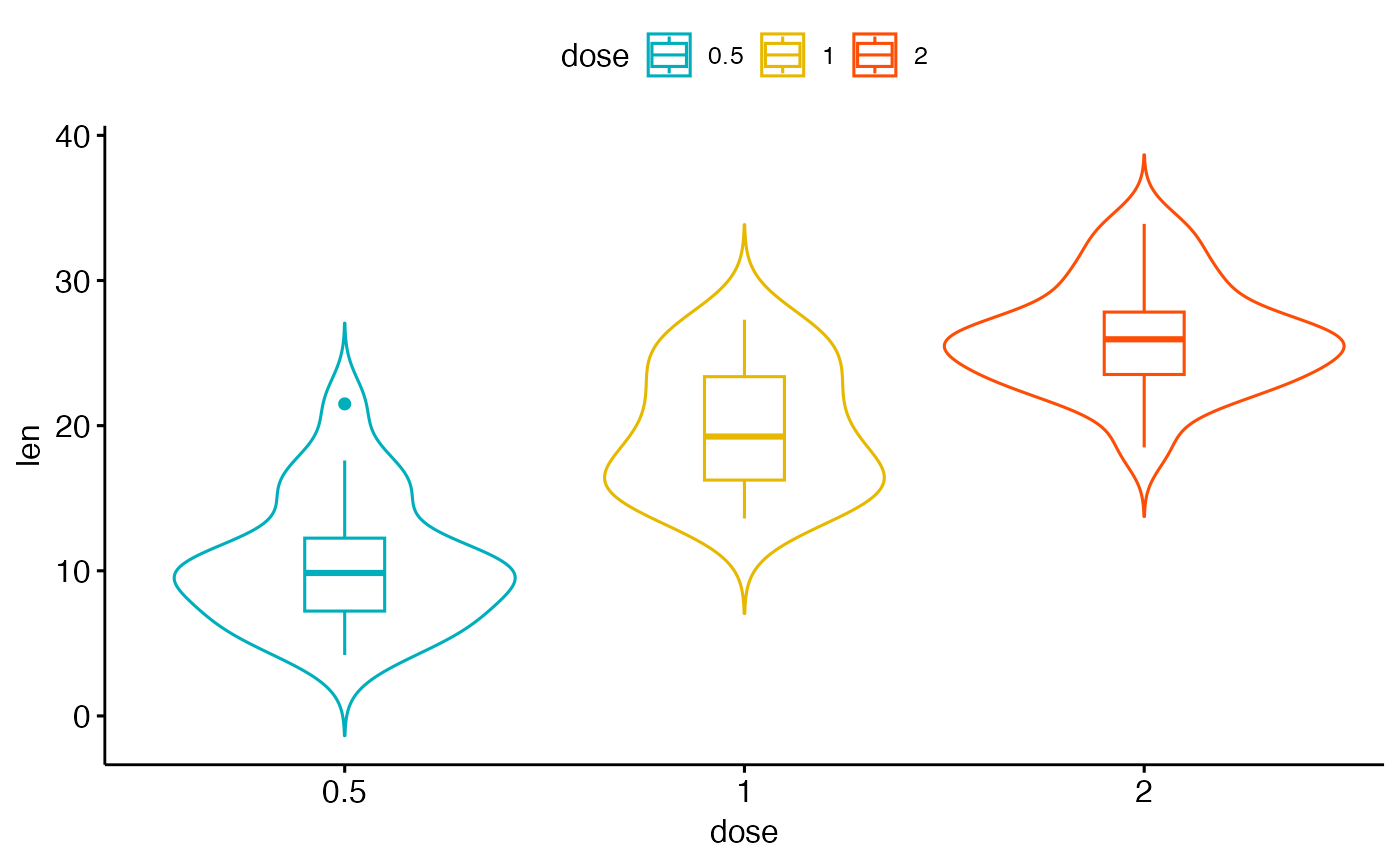# Change fill color by groups: dose
# add boxplot with white fill color
ggviolin(df, "dose", "len", fill = "dose",
palette = c("#00AFBB", "#E7B800", "#FC4E07"),